# Algebra II : Types of Numbers

## Example Questions

← Previous 1

### Example Question #1 : Types Of Numbers

Which of the following describes the number?

Possible Answers:

real, irrational

imaginary, irrational

real, rational

real, natural

Correct answer:

real, irrational

Explanation:is a real number, because you can represent it on the Cartesian coordinate plane, but it is irrational because it cannot be represented by a fraction of two integers. Natural numbers are integers greater than 0.

### Example Question #1 : Types Of Numbers

Which of the following sets of numbers contain only natural numbers.

Possible Answers: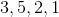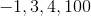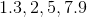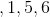Correct answer:Explanation:

Natural numbers are simply whole, non-negative numbers.

Using this definition, we see only one set of numbers within our answer choices containing only whole, non-negative numbers. Any set containing decimals or negative numbers, will violate our defintion of natural numbers and thus be an incorrect answer.

### Example Question #1 : Types Of Numbers

What is the value of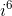?

Possible Answers: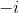Correct answer:Explanation:

There is a repeating pattern of four exponent values of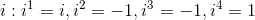.is the same as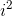.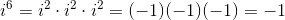### Example Question #1 : Types Of Numbers

Simplify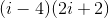.

Possible Answers: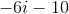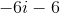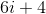Correct answer:Explanation:

Multiplying out using FOIL (First, Inner, Outer, Last) results in,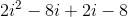.

Remember that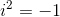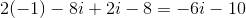### Example Question #5 : Types Of Numbers

Which of these numbers is prime?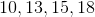Possible Answers:Correct answer:Explanation:

For a number to be prime it must only have factors of one and itself.

10 has factors 1, 2, 5, 10.

15 has factors 1, 3, 5, 15.

18 has factors 1, 2, 3, 6, 9, 18.

The only factors of 13 are 1 and 13. As such it is prime.

### Example Question #1 : Types Of Numbers

Which of the below is an irrational number?

Possible Answers: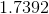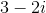Correct answer:Explanation:

Irrational numbers are defined by the fact that they cannot be written as a fraction which means that the decimals continue forever.

Looking at our possible answer choices we see,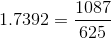is already in fraction form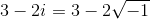which is an imaginary number but still rational.

Therefore since,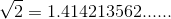we can conclude it is irrational.

### Example Question #7 : Types Of Numbers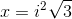Which of the following describes the type of?

Possible Answers:is real, rationalis imaginary, irrational

None of these options describe.is imaginary, rationalis real, irrational

Correct answer:is real, irrational

Explanation:

An irrational number is a number that cannot be written in fraction form. In other words a nonrepeating decimal is an irrational number.

The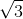is an irrational number.is a real number with a value of.

Therefore,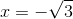. This is a real but irrational number.

### Example Question #8 : Types Of Numbers

What is the most specific classification for the x-intercepts to the equation graphed: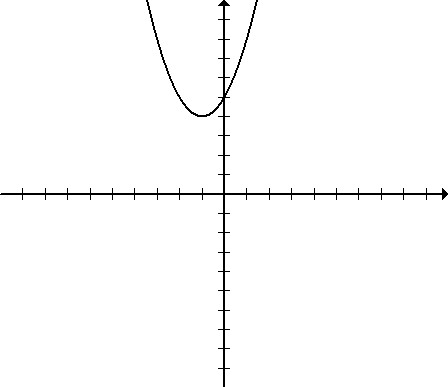Possible Answers:

integer

real

irrational

imaginary

rational

Correct answer:

imaginary

Explanation:

The graph shown never intersects with the x-axis. This means that the x-intercepts must be imaginary.

### Example Question #9 : Types Of Numbers

What is the most specific classification for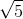Possible Answers:

irrational

rational

counting number

imaginary

natural number

Correct answer:

irrational

Explanation:

The square root of 5 is irrational since it is a non-terminating, non-repeating decimal that cannot be expressed as a fraction.

### Example Question #1 : Types Of Numbers

Which of the following is a rational number?

Possible Answers: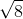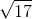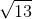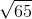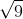Correct answer:Explanation: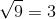A rational number is a number that can be expressed in the form p/q. in this case p=3 and q=1. The other answers are irrational because they cannot be expressed as whole numbers or fractions.

← Previous 1

### All Algebra II Resources